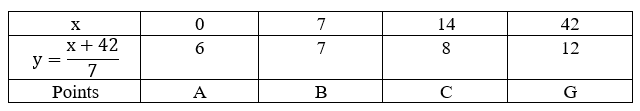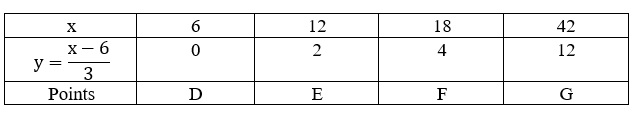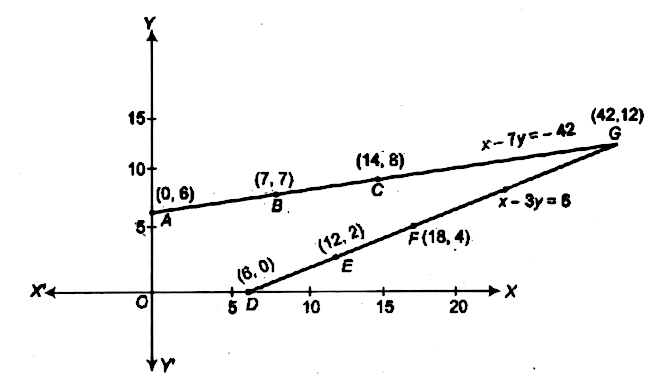Home/Class 10 Math Chapter List/3. Pair of Linear Equations in Two Variables/

# Aftab tells his daughter,Seven years ago ,I was seven times as old as you were then.Also, three years from now, I shall be three times as old as you will be.( Isn't this interesting?).Represent this situation algebraically and graphically.

## QuestionMathClass 10

Aftab tells his daughter,"Seven years ago ,I was seven times as old as you were then.Also, three years from now, I shall be three times as old as you will be".( Isn't this interesting?).Represent this situation algebraically and graphically.

See the analysis below
4.64.6## Solution

Let the present age of father be $$x$$ years and the age of daughter be $$y$$ years
Seven years ago father's age$$=(x-7)$$yr
Seven years ago daughter's age$$=(y-7)$$yr
According to the problem$$,(x-7)=7(y-7)$$
or $$x-7y=-42\cdots\cdots(i)$$
After 3 yr father's age$$=(x+3)$$yr
After 3 yr daughter's age$$=(y+3)$$yr
According to the condition given in the questions
$$x+3=3( y+3)$$
or  $$x-3y=6 \cdots \cdots (ii)$$
Hence, algebraically the situation is represented by equations
$$x-7y=-42$$
$$x-3y=6$$ where $$x$$ is the present age of father and $$y$$ is the present age of his daughter.
To find the equivalent geometric representation$$,$$ we find some points on the line representing each equation$$.$$ These solutions are given below in the table$$.$$
From $$Eq.(i),x-7y=-42$$From $$Eq.(ii),x-3y=6$$Hence, situation is represented graphically.